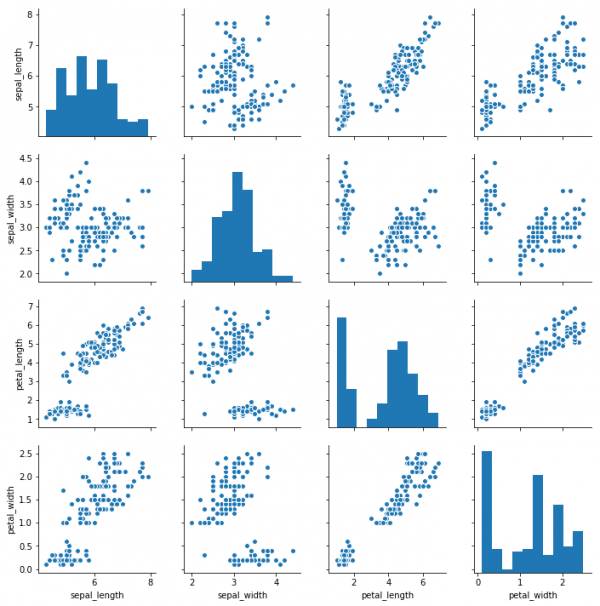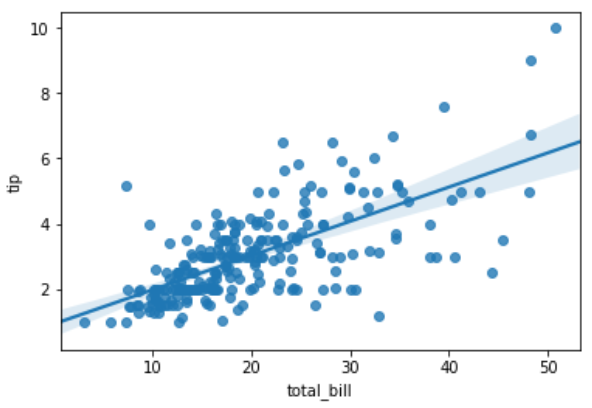# Correlation and Regression in Python

Correlation refers to some statistical relationships involving dependence between two data sets. While linear regression is a linear approach to establish the relationship between a dependent variable and one or more independent variables. A single independent variable is called linear regression whereas multiple independent variables is called multiple regression.

## Correlation

Simple examples of dependent phenomena include the correlation between the physical appearance of parents and their offspring, and the correlation between the price for a product and its supplied quantity.We take example of the iris data set available in seaborn python library. In it we try to establish the correlation between the length and the width of the sepals and petals of three species of iris flower. Based on the correlation found, a strong model could be created which easily distinguishes one species from another.

## Example

import matplotlib.pyplot as plt
import seaborn as sns
#without regression
sns.pairplot(df, kind="scatter")
plt.show()

## Output

Running the above code gives us the following result −## Linear Regression

Mathematically a linear relationship represents a straight line when plotted as a graph. A non-linear relationship where the exponent of any variable is not equal to 1 creates a curve. The functions in Seaborn to find the linear regression relationship is regplot. The below example shows its use.

## Example

import seaborn as sb
from matplotlib import pyplot as plt
plt.show()